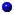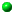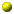## Reprints and Preprints in .pdf format

### Recent workPreprints of most of my mathematical papers written after 2005 can be found at the arXiv.

### Math papersEarly unpublished papers in hyperbolic geometry. Which have remained, for some reason, unpublished. These are pdf files of scans of old typed papers....they're from before I learned TeX. Displayed at full size they are quite readable.

1. My first paper on the hyperbolic Gauss map and its application to study hypersurfaces in hyperbolic space: Envelopes of Horospheres and Weingarten Surfaces in  Hyperbolic 3-space.
2. Another paper on a similar topic: a version of Axel Schur's Lemma, comparing arclength with chordal length for curves in hyperbolic space:  The Theorem of A. Schur in Hyperbolic Space.
3. A maple worksheet illustrating a Wankel engine.Joint papers with Richard B. Melrose:
We compute the index of a fourier integral operator defined by a contact diffeomorphism of a contact manifold. In MRL. Contact degree and the index of Fourier integral operators.
This is the long lost paper, Shrinking tubes and the d-bar Neumann problem. It's filled with tasty morsels, but it not recommeded for a single sitting. It contains a proof of the "The Boutet de Monvel-Guillemin" conjecture as well as the complete metric approach to the Bergmann kernel and a study of the d-bar Neumann problem on shrinking Grauert tubes.Joint papers with Gennadi Henkin:
We consider strict complex cobordisms and embeddability for 3d CR-manifolds. This paper includes an appendix by Bruno De Oliveira. In Proceedings of the Steklov Inst. (Corrected version of April 17, 2006) Can a good manifold come to a bad end?

This is a paper about embedding pseudoconcave manifolds and the stability of the algebra of CR-functions under deformations. In Acta Math. Stability of embeddings for pseudoconcave surfaces and their boundaries

Two local results about generically one to one analytic maps: Two lemmas in local analytic geometry

An expository paper on pseudoconcave manifolds and \$L^2\$-methods: Embedding 3-dimensional CR-manifoldsA note on the deformation theory of open Stein manifolds. In CPDE. Deformations of open Stein manifoldsNotes from lectures I gave at CIRM, in Luminy, France in June of 1999 on Toeplitz operators, index theorems and relative indices, revised in 2002 for the WHOI publication. Lectures on Indices and Relative Indices on Contact and CR-ManifoldsNotes from a lecture I gave in December 1999 at the Conference on Several Complex Variables, Hayama, Japan. Filling pseudodoncave holesA short paper correcting an error in "A relative index on the space of embeddable CR-structures, I" Ann. of Math. ErratumUnder the same cohomologial hypotheses used in Stability of embeddings for pseudoconcave surfaces and their boundaries, I prove the conjecture that, among sufficiently small deformations of the CR-structure on a strictly pseudoconvex, 3-manifold, the relative index assumes only finitely many different values. Appears in JIMJ. Geometric bounds on the relative indexA short paper on convex regions. Convex regions, shadows and the Gauss mapA short paper on subelliptic boundary conditions for the Spin-C Dirac operator on a complex manifold: A priori estimates for a subelliptic Spin-C Dirac operatorA little note on the convergence of the Neumann series in higher norms with applications to the Marchenko equation (in CPDE): The convergence of the Neumann series in higher norms.A little note comparing the finite Fourier transform to the Fourier transform (in CPAM): How well does the finite Fourier transform approximate the Fourier transform?Two papers on a careful analysis of subelliptic boundary conditions for the Spin-C Dirac operator and gluing formulae for holomorphic Euler characteristics on partitioned compact, complex manifolds (in the Annals of Math.):

Subelliptic Spin-C Dirac operators, I (revised 5/23/05) Subelliptic Spin-C Dirac operators, II (revised 11/19/07)This is part III. I extend the subelliptic boundary conditions for the Spin-C Dirac operator to Spin_C manifolds with almost complex boundaries. This leads to an Agranovich-Dynin formula and a proof of formula for the relative between two generalized Szego projectors. The Atiyah-Weinstein, on the index of Fourier integral operator defined by a contact transformation is a special case. I also get a very beautiful formula for the relative index between two classical Szego projectors defined by embeddable CR-structures on a contact 3-manifold,  (in the Annals of Math.).

Subelliptic Spin-C Dirac operators, III: The Atiyah-Weinstein conjecture.An expository account of my work on sub-elliptic boundary value problems for the Spin_C Dirac operator. In PNAS.

Subelliptic boundary conditions for Spin_C-Dirac Operators, gluing, relative indices, and tame Fredholm pairs.A short note with Jack Morava on the analytic extension of the polylogarithm functions, on the real line, as an entire family of tempered distributions. Tempering the Polylog.A paper with John Schotland, where we analyze of the "inverse" of the Laplace, and Laplace-like transforms: The Bad Truth About Laplace's Transform,  in SIAM review.

### Imaging papersMy first foray into magnetic resonance imaging. This paper is about using inverse scattering to design selective RF-pulses. (final version appears in JMR) Minimum energy pulse synthesis via the inverse scattering transformA paper on magnetic resonance imaging in inhomogeneous background fields. (appears in Inverse Problems) Magnetic Resonance Imaging in Inhomgeneous FieldsA paper the convergence properties of the hard pulse approximation for the 2x2 AKNS system (Spin domain Bloch Equation) with Jeremy Magland (in Inverse Problems) The hard pulse approximation for the 2x2 AKNS systemAn article, with Felix Wehrli, on MRI for the Elsevier Encyclopedia on Mathematical Physics Magnetic Resonance ImagingAn article, with Jeremy Magland, on finding missing uniformly spaced samples, when sampling on a polar grid Ramp sampling and projection reconstruction

### Signal Processing

• Bandwidth and Bad Driving  A short note explaining the physiological origins of the danger of driving while talking on a cell phone.

Other preprints and reprints available by sending e-mail to CL Epstein cle@math.upenn.edu